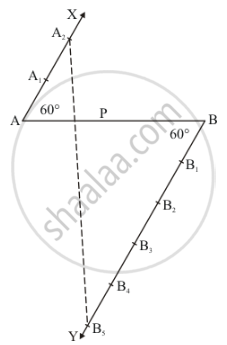Share

# Divide a Line Segment of Length 14 Cm Internally in the Ratio 2 : 5. Also, Justify Your Construction. - CBSE Class 10 - Mathematics

#### Question

Divide a line segment of length 14 cm internally in the ratio 2 : 5. Also, justify your construction.

#### Solution

Given that

Determine a point which divides a line segment of length 14cm internally in the ratio of 2:5.

We follow the following steps to construct the givenStep of construction

Step: I-First of all we draw a line segment AB = 14cm.

Step: II- We draw a ray AX making an acute angle ∠BAX = 60° with AB.

Step: III- Draw a ray BY parallel to AX by making an acute angle ∠ABY = ∠BAX.

Step IV- Mark of two points A1, A2 on AX and three points B1, B2, B3, B4, B5 on BY in such a way that AAA1 = A1A2 = B1B2 = B2B3 = B3B4 = B4B5.

Step: V- Joins A2B3 and this line intersects AB at a point P.

Thus, P is the point dividing AB internally in the ratio of 2:5

Justification:-

In ΔAA2P and ΔBB5P we have

∠A2AP = ∠PBB5          [∠ABY = ∠BAX]

And ∠APA2 = ∠BPB5    [Vertically opposite angle]

So, AA similarity criterion, we have

ΔAA2P ≈ ΔBB5P

(A A_2)/(BB_5)=(AP)(BP)

(AP)/(BP)=2/5

Is there an error in this question or solution?

#### APPEARS IN

Solution Divide a Line Segment of Length 14 Cm Internally in the Ratio 2 : 5. Also, Justify Your Construction. Concept: Division of a Line Segment.
S